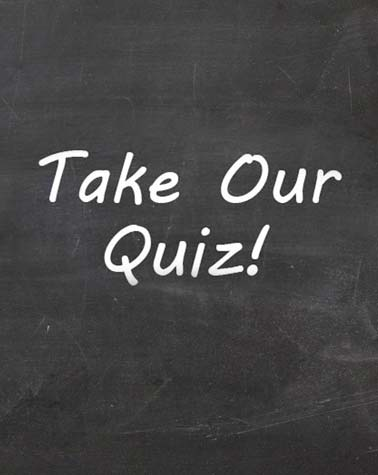# Medical Entrance Examinations Test

40 Questions | Attempts: 233
ShareSettingsFor More MCAT & ECAT MCQS Knowledge Join 👇 this group. Https://www. Facebook. Com/groups/1245646258907434/

• 1.
Which zeros are significant in the number 0.01030
• A.

The zero between the 1 and 3, and the zero after the 3 is significant.

• B.

Only the zero at the end is significant.

• C.

All of the zeros are significant.

• D.

Only the zero between the 1 and 3 is significant.

• 2.
Round the number 45 to one significant figure.
• A.

45

• B.

50

• C.

40

• D.

46

• 3.
How many significant figures should the answer have if we multiply: 5.60 x 3.2
• A.

4

• B.

2

• C.

3

• D.

1

• 4.
How many significant figures should the answer have if we divide: 100 / 0.001
• A.

2

• B.

4

• C.

3

• D.

1

• 5.
How would you write the number 200 so that you had 2 significant figures?
• A.

200.0

• B.

2 x 10^2

• C.

20.0

• D.

2.0 x 10^2

• 6.
Add 15.5m, 651.8cm, and 4291 mm
• A.

25.390

• B.

26.309

• C.

27.301

• D.

29

• 7.
Formula mass of NaCl is
• A.

57.5 amu

• B.

58.5 amu

• C.

59.5 amu

• D.

60.0 amu

• 8.
Substance which has formula mass of 133.5 amu is
• A.

MgCl2

• B.

S2Cl2

• C.

BCl3

• D.

AlCl3

• 9.
All are ionic compounds except
• A.

AgCl

• B.

HNO3

• C.

NO2

• D.

(NH4)2CO3

• 10.
Calculate the volume of  CO2 gas at STP by the combustion of 20grams of CH4.
• A.

27dm^3

• B.

28dm^3

• C.

26dm^3

• D.

25dm^3

• 11.
Nitric acid can be neutralized by any base to form a salt and water, as in the following equation: Mg(OH)2  +  2 HNO3  →  Mg(NO3)2  +  2 H­2O How much magnesium nitrate salt will be formed by the reaction of 250. g magnesium hydroxide with 250. g nitric acid?
• A.

318 g

• B.

636 g

• C.

294 g

• D.

147 g

• 12.
Unit which is not derived is
• A.

Newton

• B.

Kilogramme

• C.

Watt

• D.

Pascal

• 13.
Amount of a substance in terms of numbers is measured in
• A.

Gram

• B.

Kilogramme

• C.

Mole

• D.

Not

• 14.
A single system on which all scientists all over world agree for units of measurement is called
• A.

SI units

• B.

International System of Units

• C.

Both above

• D.

None

• 15.
One nanometer is equal to
• A.

10-6 m

• B.

10-8 m

• C.

10-9 m

• D.

10-5 m

• 16.
One kilometer is equal to how many miles?
• A.

1.6

• B.

1

• C.

0.92

• D.

0.82

• 17.
How many Dynes are there in 1 gram weight?
• A.

981

• B.

900

• C.

575

• D.

475

• 18.
1 g is equal to
• A.

10-2kg

• B.

10-3kg

• C.

10-9kg

• D.

None

• 19.
The solid angle lies in the category of
• A.

Basic units

• B.

Derived units

• C.

Supplementary units

• D.

None

• 20.
The dimension of modulus of elasticity is:
• A.

Different from that of coefficient of viscosity

• B.

The same as that of pressure

• C.

Both Above

• D.

None

• 21.
Number can be expressed in scientific notation which employ power of:
• A.

1

• B.

10

• C.

100

• D.

1000

• 22.
• A.

Work

• B.

Pressure

• C.

Energy

• D.

Torue

• 23.
The dimensions MLT-2 refer to the physical quantity.
• A.

Torque

• B.

Force

• C.

Mass

• D.

Acceleration

• 24.
Pencillin was discovered by
• A.

Fleming

• B.

Jenner

• C.

Robert Brown

• D.

Laveran

• 25.
Hydroponics is the science of growing terrestrial plants in
• A.

Laboratory

• B.

Desert

• C.

Lake

• D.

Aerated solution

## Related TopicsBack to top
×

Wait!
Here's an interesting quiz for you.